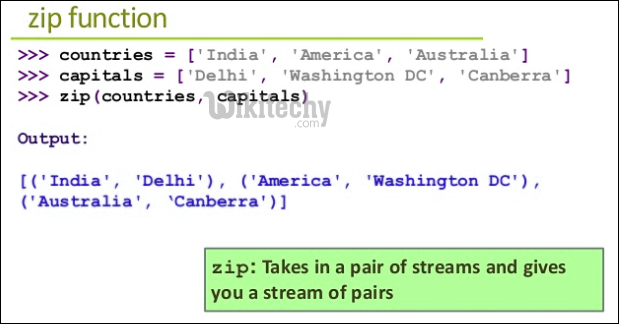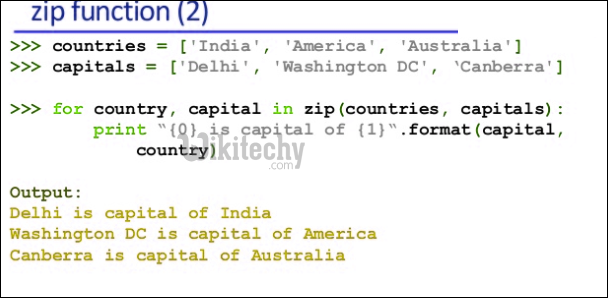# python tutorial - Python Iterate | Using Iterations in Python Effectively - learn python - python programmingLearn Python - Python tutorial - python iterations - Python examples - Python programs

Following are different ways to use iterators.

C-style approach:
This approach requires prior knowledge of total number of iterations.

python - Sample - python code :

python tutorial - Output :

```Aston
Audi
McLaren
```

Important Points:

• This style of looping is rarely used by python programmers.
• This 4-step approach creates no compactness with single-view looping construct.
• This is also prone to errors in large-scale programs or designs.
• There is no C-Style for loop in Python, i.e., a loop like for (int i=0; i<n; i++)

Use of for-in (or for each) style:

This style is used in python containing iterator of lists, dictonary, n dimensional-arrays etc. The iterator fetches each component and prints data while looping. The iterator is automatically incremented/decremented in this construct.

python - Sample - python code :

python tutorial - Output :

```Aston
Audi
McLaren
```

Indexing using Range function: We can also use indexing using range() in Python.

python - Sample - python code :

python tutorial - Output :

```Aston
Audi
McLaren
```

Enumerate:

Enumerate is built-in python function that takes input as iterator, list etc and returns a tuple containing index and data at that index in the iterator sequence. For example, enumerate(cars), returns a iterator that will return (0, cars), (1, cars), (2, cars), and so on.

python - Sample - python code :

python tutorial - Output :

```Aston
Audi
McLaren ```

Below solution also works.

python - Sample - python code :

python tutorial - Output :

```(0, 'Aston')
(1, 'Audi')
(2, 'McLaren ')```

We can also directly print returned value of enumerate() to see what it returns.

python - Sample - python code :

python tutorial - Output :

`[(0, 'Aston'), (1, 'Audi'), (2, 'McLaren ')]`

Enumerate takes parameter start which is default set to zero. We can change this parameter to any value we like. In the below code we have used start as 1.

python - Sample - python code :

python tutorial - Output :

```(1, 'Aston')
(2, 'Audi')
(3, 'McLaren ')```

enumerate() helps to embed solution for accessing each data item in iterator and fetching index of each data item.

Looping extensions:

i) Two iterators for a single looping construct: In this case, a list and dictionary are to be used for each iteration in a single looping block using enumerate function. Let us see example.

python - Sample - python code :

python tutorial - Output :

```Car: Aston Price: 570000\$
Car: Audi Price: 68000\$
Car: McLaren Price: 450000\$
Accessory: GPS kit Price: 890000\$
Accessory: Car repair-tool kit Price: 4500\$
```

ii) zip function (Both iterators to be used in single looping construct):
This function is helpful to combine similar type iterators(list-list or dict- dict etc) data items at ith position. It uses shortest length of these input iterators. Other items of larger length iterators are skipped. In case of empty iterators it returns No output.For example, the use of zip for two lists (iterators) helped to combine a single car and its required accessory.

python - Sample - python code :

python tutorial - Output :

```Car: Aston, Accessory required: GPS
Car: Audi, Accessory required: Car Repair Kit
Car: McLaren, Accessory required: Dolby sound kit```

The reverse of getting iterators from zip function is known as unzipping using “*” operator.

Use of enumerate function and zip function helps to achieve an effective extension of iteration logic in python and solves many more sub-problems of a huge task or problem.python - Sample - python code :

python tutorial - Output :

```('Aston', 'Audi', 'McLaren')
('GPS', 'Car Repair', 'Dolby sound kit')
```

Wikitechy tutorial site provides you all the learn python , python code , python script , python windows , python mysql , django python , python django , python compiler , jython , python interpreter , what is python , python join , python input , python modules , python editor , python examples , python pdf , python documentation